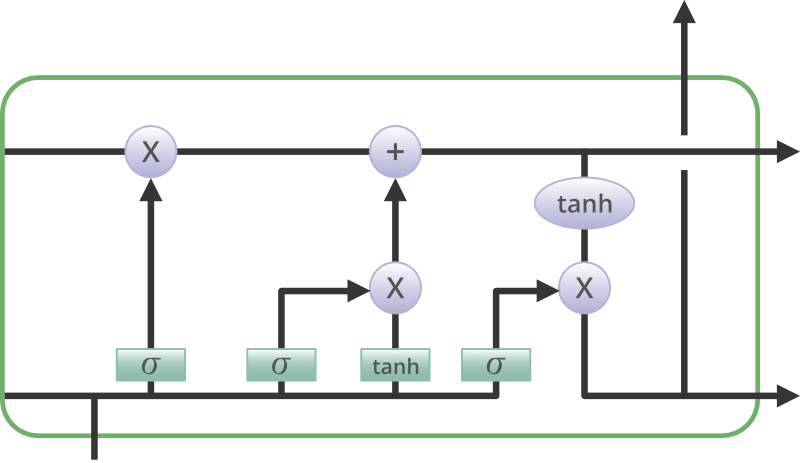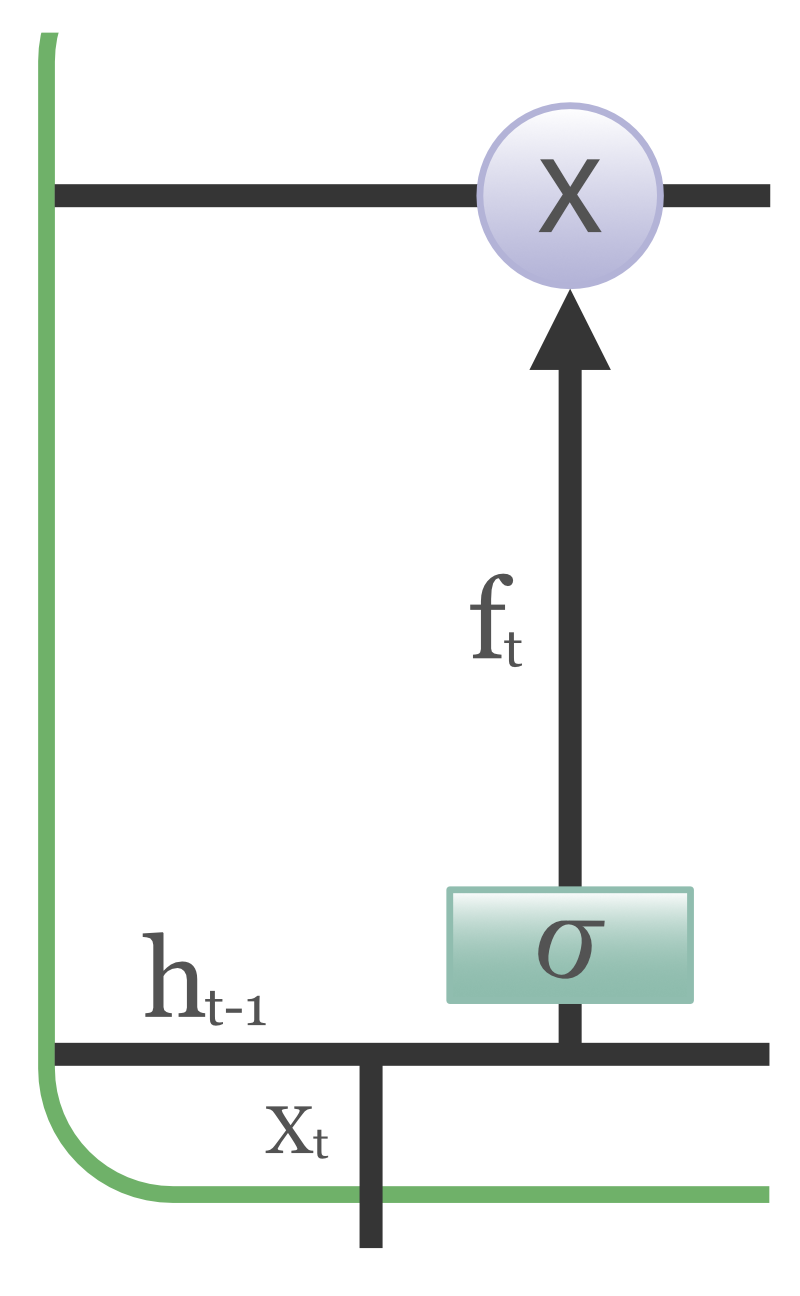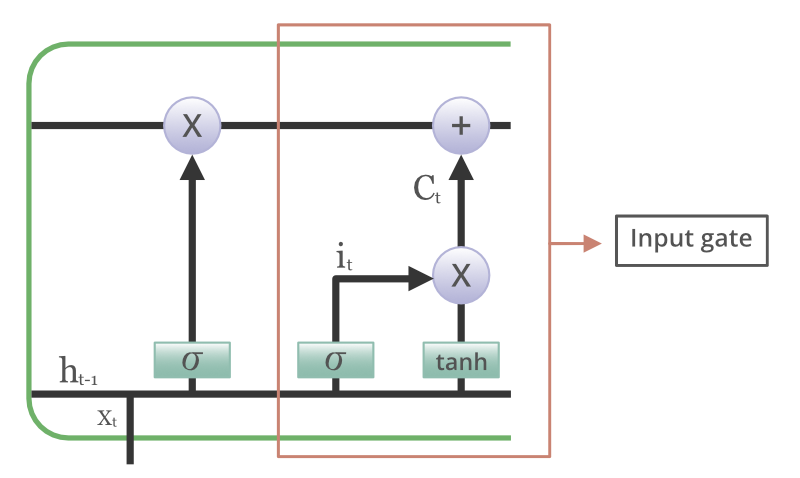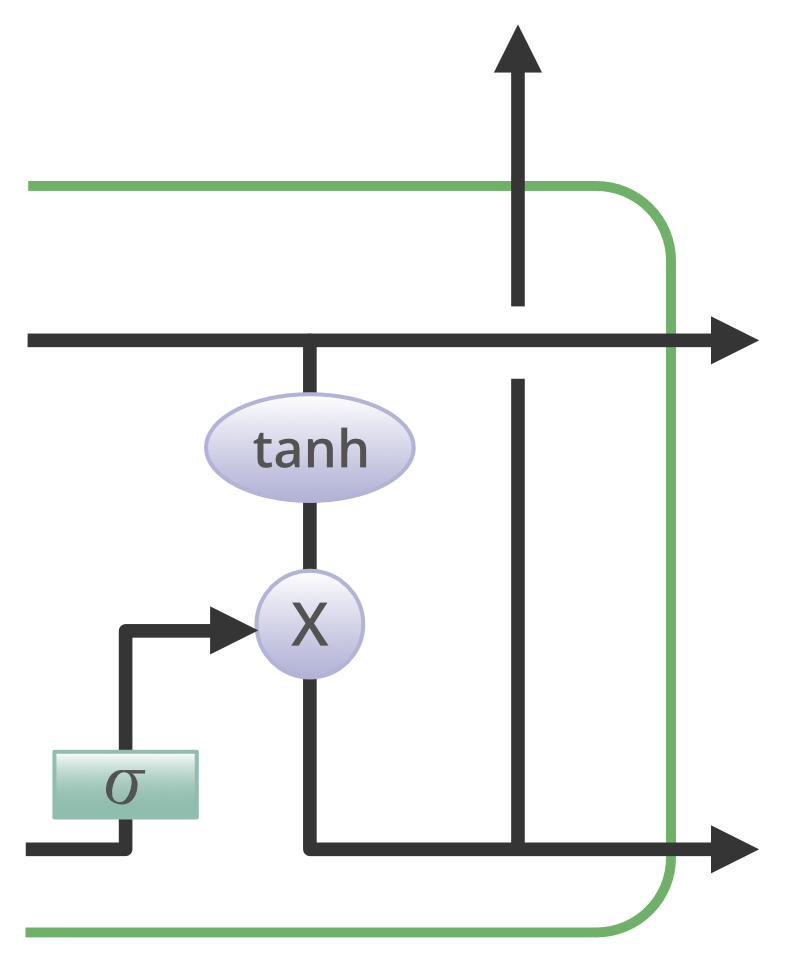GeeksforGeeks App
Open AppBrowser
Continue

# Deep Learning | Introduction to Long Short Term Memory

Long Short Term Memory is a kind of recurrent neural network. In RNN output from the last step is fed as input in the current step. LSTM was designed by Hochreiter & Schmidhuber. It tackled the problem of long-term dependencies of RNN in which the RNN cannot predict the word stored in the long-term memory but can give more accurate predictions from the recent information. As the gap length increases RNN does not give an efficient performance. LSTM can by default retain the information for a long period of time. It is used for processing, predicting, and classifying on the basis of time-series data.

Long Short-Term Memory (LSTM) is a type of Recurrent Neural Network (RNN) that is specifically designed to handle sequential data, such as time series, speech, and text. LSTM networks are capable of learning long-term dependencies in sequential data, which makes them well suited for tasks such as language translation, speech recognition, and time series forecasting.

A traditional RNN has a single hidden state that is passed through time, which can make it difficult for the network to learn long-term dependencies. LSTMs address this problem by introducing a memory cell, which is a container that can hold information for an extended period of time. The memory cell is controlled by three gates: the input gate, the forget gate, and the output gate. These gates decide what information to add to, remove from, and output from the memory cell.

The input gate controls what information is added to the memory cell. The forget gate controls what information is removed from the memory cell. And the output gate controls what information is output from the memory cell. This allows LSTM networks to selectively retain or discard information as it flows through the network, which allows them to learn long-term dependencies.

LSTMs can be stacked to create deep LSTM networks, which can learn even more complex patterns in sequential data. LSTMs can also be used in combination with other neural network architectures, such as Convolutional Neural Networks (CNNs) for image and video analysis.

### Structure Of LSTM:

LSTM has a chain structure that contains four neural networks and different memory blocks called cells.Information is retained by the cells and the memory manipulations are done by the gates. There are three gates –

1. Forget Gate: The information that is no longer useful in the cell state is removed with the forget gate. Two inputs x_t (input at the particular time) and h_t-1 (previous cell output) are fed to the gate and multiplied with weight matrices followed by the addition of bias. The resultant is passed through an activation function which gives a binary output. If for a particular cell state the output is 0, the piece of information is forgotten and for output 1, the information is retained for future use. The equation for the forget gate is:

f_t = σ(W_f · [h_t-1, x_t] + b_f)
where:

• W_f represents the weight matrix associated with the forget gate.
• [h_t-1, x_t] denotes the concatenation of the current input and the previous hidden state.
• b_f is the bias with the forget gate.
• σ is the sigmoid activation function.2. Input gate: The addition of useful information to the cell state is done by the input gate. First, the information is regulated using the sigmoid function and filter the values to be remembered similar to the forget gate using inputs h_t-1 and x_t. Then, a vector is created using tanh function that gives an output from -1 to +1, which contains all the possible values from h_t-1 and x_t. At last, the values of the vector and the regulated values are multiplied to obtain the useful information. The equation for the input gate is:

i_t = σ(W_i · [h_t-1, x_t] + b_i)

Ĉ_t = tanh(W_c · [h_t-1, x_t] + b_c)

C_t = f_t ⊙ C_t-1 + i_t ⊙ Ĉ_t

where

•  ⊙ denotes element-wise multiplication
• tanh is tanh activation function3. Output gate: The task of extracting useful information from the current cell state to be presented as output is done by the output gate. First, a vector is generated by applying tanh function on the cell. Then, the information is regulated using the sigmoid function and filter by the values to be remembered using inputs h_t-1 and x_t. At last, the values of the vector and the regulated values are multiplied to be sent as an output and input to the next cell. The equation for the output gate is:

o_t = σ(W_o · [h_t-1, x_t] + b_o)1. Long-term dependencies can be captured by LSTM networks. They have a memory cell that is capable of long-term information storage.
2. In traditional RNNs, there is a problem of vanishing and exploding gradients when models are trained over long sequences. By using a gating mechanism that selectively recalls or forgets information, LSTM networks deal with this problem.
3. LSTM enables the model to capture and remember the important context, even when there is a significant time gap between relevant events in the sequence. So where understanding context is important, LSTMS are used. eg. machine translation.

1. Compared to simpler architectures like feed-forward neural networks LSTM networks are computationally more expensive. This can limit their scalability for large-scale datasets or constrained environments.
2. Training LSTM networks can be more time-consuming compared to simpler models due to their computational complexity. So training LSTMs often requires more data and longer training times to achieve high performance.
3. Since it is processed word by word in a sequential manner, it is hard to parallelize the work of processing the sentences.

Some of the famous applications of LSTM includes:

1. Long Short-Term Memory (LSTM) is a powerful type of Recurrent Neural Network (RNN) that has been used in a wide range of applications. Here are a few famous applications of LSTM:
2. Language Modeling: LSTMs have been used for natural language processing tasks such as language modeling, machine translation, and text summarization. They can be trained to generate coherent and grammatically correct sentences by learning the dependencies between words in a sentence.
3. Speech Recognition: LSTMs have been used for speech recognition tasks such as transcribing speech to text and recognizing spoken commands. They can be trained to recognize patterns in speech and match them to the corresponding text.
4. Time Series Forecasting: LSTMs have been used for time series forecasting tasks such as predicting stock prices, weather, and energy consumption. They can learn patterns in time series data and use them to make predictions about future events.
5. Anomaly Detection: LSTMs have been used for anomaly detection tasks such as detecting fraud and network intrusion. They can be trained to identify patterns in data that deviate from the norm and flag them as potential anomalies.
6. Recommender Systems: LSTMs have been used for recommendation tasks such as recommending movies, music, and books. They can learn patterns in user behavior and use them to make personalized recommendations.
7. Video Analysis: LSTMs have been used for video analysis tasks such as object detection, activity recognition, and action classification. They can be used in combination with other neural network architectures, such as Convolutional Neural Networks (CNNs), to analyze video data and extract useful information.
My Personal Notes arrow_drop_up